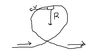# Topic 2.4: Centripetal Forces Flashcards Preview

## IB SL Physics > Topic 2.4: Centripetal Forces > Flashcards

Flashcards in Topic 2.4: Centripetal Forces Deck (18):
1

## A ball moves along the inside of a horizontal semi-circular ring as shown. The diagram is a view from above.Which arrow represents the direction of the average force on the ball?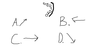2

3

## A car moves from X to Y along a semicircular path. The radius of the path is 250m and the time taken to complete the trip is 50s. Which of the following correctly shows the magnitude of the average velocity and the magnitude of the average speed?                            Average Velocity                       Average SpeedA.                         10 ms-1                                         10 ms-1B.                         10 ms-1                                          10 ms-1C.                         16 ms-1                                          10 ms-1D.                         16 ms-1                                           16 ms-14

## A particle P is moving anti-clockwise with constant speed in a horizontal circle. Which diagram correctly shows the direction of the velocity v and acceleration a of the particle P in the position shown?5

## A brick is placed on the surface of a flat horizontal disc as shown in the diagram below. The disc is rotating at constant speed about a vertical axis through its centre. The brick does not move relative to the disc.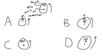6

7

## The Moon orbits the Earth. Which of the following diagrams correctly represents the force(s) acting on the Moon?8

9

## The Moon orbits the Earth in a nearly circular orbit at constant speed as shown. Which of the following diagrams correctly shows the force(s) acting on the Moon in the position shown above?10

## A ball is swinging in a horizontal circle as shown in the diagram below. Which one of the following diagrams best shows the forces acting on the ball when it is at the position shown above?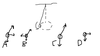11

12

13

## A point mass is moving in a horizontal circle with a velocity of constant magnitude v. At one particular time, the mass is at P. A short time later, the mass is at Q, as shown below. Which vector diagram correctly shows the change in velocity ∆v of the mass during this time?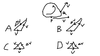14

## Which of the following diagrams represents the direction of the centripetal force F acting on a car travelling in a circular path?15

## Two points P and Q are at distances r and 2r respectively from the centre of a compact disc (CD) as shown. When the disc is rotating about its centre, the ratio of the acceleration at P/acceleration at Q  is  A. 1/2 B. 1 C. 21/2 D. 2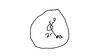16

17

18

## In a fairground ride, a car of mass M travels on rails around a vertical loop of effective radius R. At the top of the loop, the speed of the car is v. The car stays in contact with the rails, as shown below. The acceleration of free fall is g. Which of the following is the correct expression for the force that the rails exert on the car?A. (Mv2/R)- MgB. Mv2/RC. MgD. (Mv2/R)+ Mg